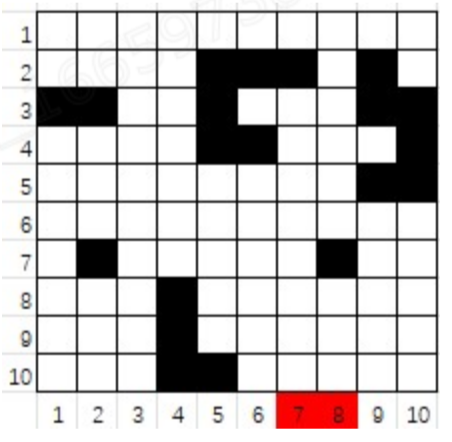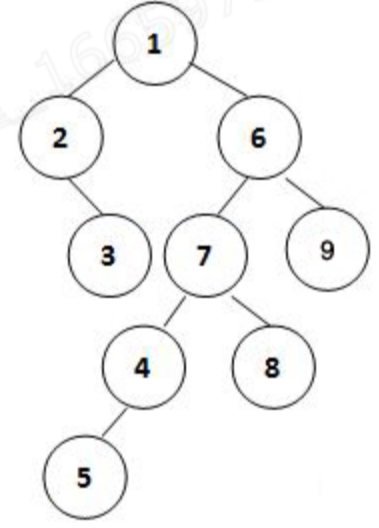A-1 Trap

A robot is designed to move on a map from South toward North. When some obstacle is encountered, the robot can only turn toward West and moves until it can turn toward North and continue.

Given a squared map with n×n blocks, a robot can start from any position below the bottom line (South) and its target is to reach the top line (North). (By the way, kindly remind you that the left-hand-side of the map is West and the right-hand-side is East.)

If some obstacles are placed in the map, the robot might get trapped if it starts from certain positions and can never reach the North line. For example, in the situation given by the following figure, the robot will be trapped if it starts from either position 7 or 8.Your job is to point out those starting positions which will get the robot trapped.

Note: it is assumed that a robot can move out of the map boundary, and all the blocks around the map are open, without any obstacle. Hence if the robot starts from any position out of the West or East boundary, it can certainly reach North without any problem. Therefore we only have to consider the positions between the West and East boundaries (e.g. the positions from 1 to 10 below the South line in the above figure). Besides, as long as the robot can reach the North line, it is considered successful even of it ends up at out of the boundary (e.g. the robot will have to move out of the map if starts from either the positions 1 or 2, but it can still reach the North line).

Input Specification:

Each input file contains one test case. Each case starts from a positive integer n (≤100) in a line, which is the size of the map. Then n lines follow, each contains n characters, which are either 0 for an open block, or 1 for a block with obstacle. The first line corresponds to the North boundary and the last line the South.

Output Specification:

Output in a line all the starting positions which can get the robot trapped, in increasing order. The positions are indexed from West to East, starting from 1. It is guaranteed that there is at least one output.

All the numbers must be separated by 1 space, and there must be no extra space at the beginning or the end of the line.

Sample Input:

``````10
0000000000
0000111010
1100100011
0000110001
0000000011
0000000000
0100000100
0001000000
0001000000
0001100000``````

Sample Output:
`7 8`

``````#include <bits/stdc++.h>
using namespace std;
bool f(const vector<string>& g, int i, int j, int n) {
if (i == 0 || j == 0) return 1;
if (g[i-1][j] == '0') return f(g, i-1, j, n);
else if (g[i][j-1] == '0') return f(g, i, j-1, n);
return 0;
}
int main() {
int n;
cin >> n;
vector<string> g(n+1, string(n, '0'));
for (int i = 0; i < n; ++i) {
cin >> g[i];
}
bool b = 0;
for (int j = 0; j < n; ++j) {
if (!f(g, n, j, n)) {
if (b == 1) cout<<' ';
cout<<j+1;
b=1;
}
}
return 0;
}``````

A-2 Queue Using Two Stacks

A queue (FIFO structure) can be implemented by two stacks (LIFO structure) in the following way:

Start from two empty stacks s1 and s2

When element e is enqueued, it is actually pushed onto s1

When we are supposed to dequeue, s2  is checked first. If s2  is empty, everything in s1 will be transferred to s2 by popping from s1  and immediately pushing onto s2. Then we just pop from s

2  -- the top element of s2 must be the first one to enter s1, thus is the first element that was enqueued.

Assume that each operation of push or pop takes 1 unit of time. You job is to tell the time taken for each dequeue.

Input Specification:

Each input file contains one test case. For each case, the first line gives a positive integer N (≤10^3 ), which are the number of operations. Then N lines follow, each gives an operation in the format

`Operation Element`
where `Operation` being I represents enqueue and O represents dequeue. For each I, `Element` is a positive integer that is no more than 10^6. No Element is given for O operations.

It is guaranteed that there is at least one O operation.

Output Specification:

For each dequeue operation, print in a line the dequeued element and the unites of time taken to do this dequeue. The numbers in a line must be separated by 1 space, and there must be no extra space at the beginning or the end of the line.

In case that the queue is empty when dequeue is called, output in a line ERROR instead.

Sample Input:

``````10
I 20
I 32
O
I 11
O
O
O
I 100
I 66
O``````

Sample Output:

``````20 5
32 1
11 3
ERROR
100 5``````

``````#include <bits/stdc++.h>
using namespace std;
int main() {
int n;
cin >> n;
stack<int> s1, s2;
for (int i = 0; i < n; ++i) {
char op;
cin >> op;
if (op == 'I') {
int ele;
cin >> ele;
s1.push(ele);
} else {
if (s1.empty() && s2.empty()) cout<<"ERROR";
else {
if (!s2.empty()) {
cout << s2.top() << ' ' << "1";
s2.pop();
} else {
int cnt = s1.size() * 2 + 1;
while (!s1.empty()) {
s2.push(s1.top());
s1.pop();
}
cout << s2.top()<< ' ' << cnt;
s2.pop();
}
}
if (i != n-1) cout<<endl;
}

}
return 0;
}``````

A-3 Rank of Binary Tree

Here we define the rank of a binary tree as n1×n2 /n0 where ni  is the number of nodes of degree i for i=0,1,2.

For example, given a tree shown by the figure, its rank is 2×3/4=1.5.Given the inorder and preorder traversal sequences of a binary tree, you are supposed to calculate its rank.

Input Specification:

Each input file contains one test case. For each case, the first line contains a positive integer N (≤20), which is the total number of nodes in the tree. Then given in the following 2 lines are the inorder and preorder traversal sequences of the tree, respectively. All the keys in the tree are distinct positive integers in the range of int.

Output Specification:

For each case, print in a line the way we calculate the rank, and the integer part of the rank. The format is:

`n1 * n2 / n0 = rank`

Sample Input:

``````9
2 3 1 5 4 7 8 6 9
1 2 3 6 7 4 5 8 9``````

Sample Output:
`2 * 3 / 4 = 1`

``````#include <bits/stdc++.h>
using namespace std;
int n0 = 0, n1 = 0, n2 = 0;
unordered_map<int, int> idx;
vector<int> in, pre;
struct Node {
Node *left, *right;
int val;
Node(int v){val = v;}
};
Node* buildTree(int pl, int pr, int il, int ir) {
if (pl > pr) return nullptr;
int proot = pl;
int iroot = idx[pre[proot]];
Node* root = new Node(pre[proot]);
int slt = iroot - il;//size of left subtree
root->left = buildTree(pl+1, pl+slt, il, iroot-1);
root->right = buildTree(pl+slt+1, pr, iroot+1, ir);
return root;
}
void nCounter(Node* node) {
if (!node) return;
if (!node->left && !node->right) {
++n0;
return;
}
nCounter(node->left);
nCounter(node->right);
if (node->left) {
if (node->right) {
++n2;
} else {++n1;}
} else if (node->right) {
++n1;
}
}
int main() {
int n;
cin >> n;
in.resize(n); pre.resize(n);
for (int i = 0; i < n; ++i) cin >> in[i];
for (int i = 0; i < n; ++i) cin >> pre[i];
for (int i = 0; i < n; ++i) idx[in[i]] = i;
auto root = buildTree(0, n-1, 0, n-1);
nCounter(root);
printf("%d * %d / %d = %d", n1, n2, n0, n1*n2/n0);
return 0;
}``````

A-4 Big Number

How to generate a big number of N digits randomly? One way is to find N kids, give each one a card with one's index written on one side (hence it is assumed that the kids are indexed from 1 to N), and ask them to write down a 1-digit number randomly on the other side. Then let the kids pin their digits in a line, on the wall, one by one in ascending order of their indices.

However, it's very difficult to let hundreds of thousands of kids to follow the order. The result is that we have cards pinned randomly all over the wall, some even show the wrong sides. For example, if the 23rd kid has written down 8, we are supposed to find the number 8 on the wall. But instead we might find 23... Your job is to rearrange these cards so that we can obtain the big number as required.

Input Specification:

Each input file contains one test case. For each case, the first line gives a positive integer N (≤10^5). Then N lines follow, each describes a card in the format n1 n2 where the two numbers are the numbers written on the two sides of a card.

Output Specification:

For each test case, print in a line the N-digit number as required. That is, print the digits written by the kids in ascending order of their indices. In case that there are 1-digit numbers written on both sides, it would be hard to tell which one is the index and which one is the number written by the kid. Hence the solution may not be unique. In this case, just output the smallest resulting number.

It is guaranteed that a solution exists.

Sample Input:

``````12
7 11
8 9
3 1
2 12
4 6
10 0
5 1
2 5
6 8
1 4
7 2
9 3``````

Sample Output:
`359114268072`

``````2
1 2
1 2``````

``````#include <bits/stdc++.h>
using namespace std;
int n;
void dfs(int cur, vector<int>& tv, vector<int>& res, set<pair<int,int>>& cap) {
//printf("\ndfs:cur=%d", cur);
if (cur == 10 || cur > n) {
res = min(res, tv);
return;
}
for (int i = 0; i < 10; ++i) {
if (cur==1&&i==0) continue;
if (cap.count({cur, i})) {
cap.erase({cur, i});
tv[cur] = i;
dfs(cur+1, tv, res, cap);
cap.emplace(cur, i);
}
if (cap.count({i, cur})) {
cap.erase({i, cur});
tv[cur] = i;
dfs(cur+1, tv, res, cap);
cap.emplace(i, cur);
}
}

}
int main() {
cin >> n;
set<pair<int,int>> cap;
vector<int> v(n+1);//storage: index >= 10
for (int i = 0; i < n; ++i) {
int a, b;
cin >> a >> b;
if (a >= 10) {
v[a] = b;
} else if (b >= 10) {
v[b] = a;
} else {
if (cap.count({a, b})) cap.emplace(b, a);
else cap.emplace(a, b);
}
}
vector<int> res(10, 0x3f3f3f3f);
vector<int> tv(10, 0x3f3f3f3f);//temp vector
dfs(1, tv, res, cap);
for (int i = 1; i < 10 && i <= n; ++i) {
cout<<res[i];
}
for (int i = 10; i <= n; ++i) cout<<v[i];
return 0;
}``````